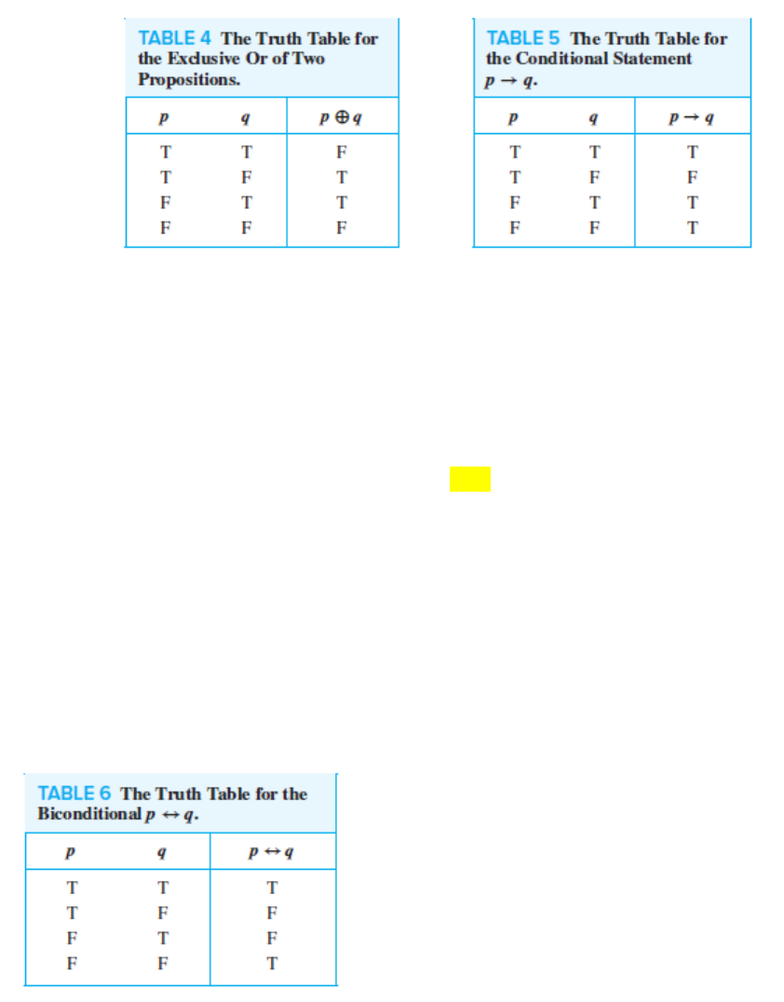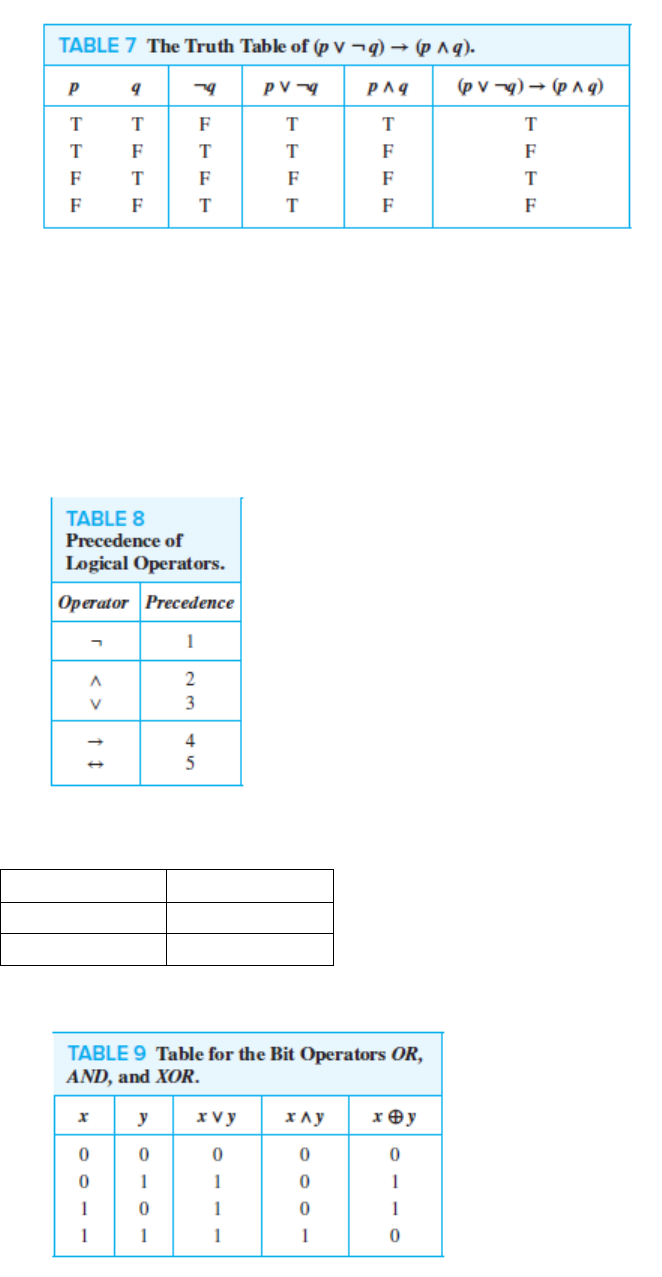Textbook Notes (280,000)
US (110,000)
COEN (10)
Chapter 1.1, 1.3

# COEN 19 Chapter Notes - Chapter 1.1, 1.3: Propositional Calculus, Logical Connective, Boolean Data Type

Department
Computer Engineering
Course Code
COEN 19
Professor
Levinson
Chapter
1.1, 1.3

This preview shows pages 1-2. to view the full 8 pages of the document.COEN 19 | 09/24/2019
Sect. 1.1
Propositions
A declarative sentence (declares a fact) that is either T/F, but not both.
e.g.
1. New York is capital of USA. | False proposition
2. 1 + 1 = 2 | True proposition
3. What time is it? | Not a proposition b/c not declarative
4. x + y = z | neither true or false
propositional variables (sentential variables): letters or variables that represent
propositions. (p, q, r, s)
Truth Value: T/F
Atomic propositions: Propositions that cannot be expressed in terms of simpler
propositions.
propositional calculus or propositional logic: area of logic that deals with propositions.
Compound propositions: new propositions made by using logical operators to combine one
or more propositions.
The notation for the negation operator is not standardized. ¬p and p bar are
common notations to express the negation of p, other notations you might see are p, p,
p, Np, and !p.
Connectives: logical operators used to form new propositions from two or more existing
propositions.
The use of the connective or in a disjunction corresponds to an inclusive or. A disjunction is
true when at least one of the two propositions is true.
The exclusive or of p and q is true when exactly one of p and q is true; it is false when both p
and q are true (and when both are false).

Only pages 1-2 are available for preview. Some parts have been intentionally blurred.If, Then Statements (Conditional Statements)
The statement p → q is a conditional statement because p → q asserts that q is true on the
condition that p holds. A conditional statement is also an implication.
e.g. If I am elected president, then I will lower taxes. | This is only false if the elected candidate lies on
their campaign promise.
Converse, Contrapositives, & Inverse
q → p is the converse of p → q.
The contrapositive of p → q is ¬q → ¬p. | Note: only the contrapositive always has the same
truth value as p q
The ¬p → ¬q is the inverse of p → q.
When two compound propositions always have the same truth values, regardless of the
truth values of its propositional variables, we call them equivalent.
If & Only If (Biconditionals)
p q is true when both p q and q p are true and is false otherwise.
Truth Tables of Compound Propositions
e.g. (p !q) (p q)
.

Unlock to view full version

Only pages 1-2 are available for preview. Some parts have been intentionally blurred.Precedence of Logical Operators
1. Not
2. And
3. Or
4. If, then
5. If & Only If
Logic & Bit Operations
Truth Value
Bit
T
1
F
0
Boolean variable: its value is either T/F.